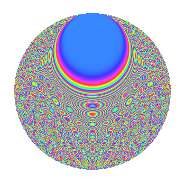# Properties

 Label 1050.2.bvLevel 1050 Weight 2 Character orbit bv Rep. character $$\chi_{1050}(73,\cdot)$$ Character field $$\Q(\zeta_{60})$$ Dimension 640 Sturm bound 480

# Related objects

## Defining parameters

 Level: $$N$$ = $$1050 = 2 \cdot 3 \cdot 5^{2} \cdot 7$$ Weight: $$k$$ = $$2$$ Character orbit: $$[\chi]$$ = 1050.bv (of order $$60$$ and degree $$16$$) Character conductor: $$\operatorname{cond}(\chi)$$ = $$175$$ Character field: $$\Q(\zeta_{60})$$ Sturm bound: $$480$$

## Dimensions

The following table gives the dimensions of various subspaces of $$M_{2}(1050, [\chi])$$.

Total New Old
Modular forms 3968 640 3328
Cusp forms 3712 640 3072
Eisenstein series 256 0 256

## Trace form

 $$640q - 24q^{5} + 12q^{7} + O(q^{10})$$ $$640q - 24q^{5} + 12q^{7} - 12q^{10} - 8q^{15} - 80q^{16} + 72q^{22} - 72q^{23} + 16q^{25} + 32q^{28} + 160q^{29} - 32q^{30} - 12q^{33} + 80q^{35} + 160q^{36} + 16q^{37} + 48q^{38} - 4q^{42} - 112q^{43} + 24q^{47} + 144q^{53} + 16q^{57} - 36q^{58} + 240q^{59} - 32q^{65} + 48q^{67} - 116q^{70} + 24q^{73} + 48q^{75} + 32q^{78} - 24q^{80} - 80q^{81} + 192q^{82} - 16q^{85} - 60q^{87} - 4q^{88} - 16q^{92} + 32q^{93} - 104q^{95} - 16q^{98} + O(q^{100})$$

## Decomposition of $$S_{2}^{\mathrm{new}}(1050, [\chi])$$ into newform subspaces

The newforms in this space have not yet been added to the LMFDB.

## Decomposition of $$S_{2}^{\mathrm{old}}(1050, [\chi])$$ into lower level spaces

$$S_{2}^{\mathrm{old}}(1050, [\chi]) \cong$$ $$S_{2}^{\mathrm{new}}(175, [\chi])$$$$^{\oplus 4}$$$$\oplus$$$$S_{2}^{\mathrm{new}}(350, [\chi])$$$$^{\oplus 2}$$$$\oplus$$$$S_{2}^{\mathrm{new}}(525, [\chi])$$$$^{\oplus 2}$$

## Hecke Characteristic Polynomials

There are no characteristic polynomials of Hecke operators in the database